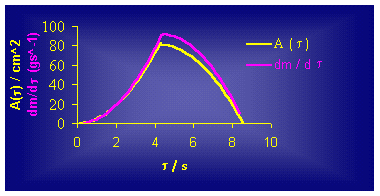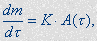VISUALIZATION OF THE REACTION ZONE SPREADING FOR AN ALUMOTHERMIC (REDOX) REACTION

Liljana Stefanovska

Faculty of Technology and Metallurgy, P.O. Box 580, Skopje, Macedonia

liljana@ereb1.mf.ukim.edu.mk

Toma Grcev

Faculty of Technology and Metallurgy, P.O. Box 580, Skopje, Macedonia

toma@ereb1.mf.ukim.edu.mk

Sonja Gegovska-Zajkova

Faculty of Electrical Engineering, P.O. Box 574, Skopje, Macedonia

szajkova@cerera.etf.ukim.edu.mk

ABSTRACT. Redox reactions between small solid particles (Me1/Me2 – oxide) are very fast exothermic and autocatalytical reactions, which take place at the solid/liquid interface. An attempt to correlate the experimentally obtained data for the alumothermic (Al/Fe3O4) reaction with several mathematical (geometrical) models for reaction zone spreading was made in this paper.

# INTRODUCTION

The processes in the redox systems, such as Me1/Me2 oxides, are basically very fast and they produce a large quantity of heat, which means that they are very high-temperature reactions. Besides we should say that these redox processes start in a solid phase (mixture of small particles of a specific metal oxide and the metal particles of the reducence) after the initiation of the reaction, the reaction continues to take place at the liquid/solid interface, during which, the new created liquid phase gravitationally falls down (moves) to the bottom of the reaction vessel due to its greater density.

One of the most common redox reactions of this type is the alumothermic reaction (ATR), where the role of an oxydance is taken over by iron oxides, such as Fe3O4, and the role of reducance - small particles of elementary alumina (Al).

An attempt to correlate the rate of the alumothermic redox reaction Al/Fe3O4 to the shape (cylinder or cone) and geometric parameters of the reaction vessel is made in this study. This reaction starts with a central initiation of the reaction mixture (small solid particles) by an electric spark and its termination occurs in several seconds.

# EXPERIMENTAL

The rate of the ATR (Al/Fe3O4) was tested in cylindrical and/or conic ceramic vessels where the quantity of reaction mixture depends on the size of the vessel. The available data in the known literature for this type of reactions, as well as our preliminary investigations, pointed out the dominant influence of the reaction vessel’s geometry and therefore its dimensions were varied regarding the diameter and the high of the vessel. Meanwhile, it was found that the time of the ATR completion, due to the very high rates of ATR (ranged between 2 and 11 s), as well as the inability to stop the process in a certain moment before the ending, the obtained results refer only to the dependence of the total reaction time on the reaction vessel’s geometry.

# RESULTS AND DISCUSSION

A part of the obtained experimental results are shown on Figure 1.Figure 1.

It is obvious that the time for finishing the reaction tr is linearly dependent on the diameter of the reaction vessel (for a constant high), as well as on the high of the vessel (at a constant diameter of the vessel). Using the regress analysis, according to the method of smallest squares, with a high coefficient of correlation (r > 0.98), these results can be represented with a great accuracy by the following equations

D = b tr           - for constant height of the reaction vessel, and

H = b’tr           - for constant diameter of the reaction vessel.

It should be mentioned that b and b’ are rate constants for a horizontal or i.e. vertical spreading of the reaction zone, respectively. The determined values of these constants point out that the constant b is a function of H (the height of the reacting vessel), and the constant b’ is a function of D (the diameter of the reaction vessel). On the other hand, it was shown that the determined values for b are two to three times smaller than those for b’, which points that the rate of horizontal spreading of the reaction zone is significantly slower than the vertical one and can be the rate determining step.

Analysing to the obtained data, the following relations can be done:The relation (3) clearly points out that the spreading of the reaction zone (b/b’) fairly good follows the geometry of the reaction vessel  (D/H). This type of dependence is obtained for both cylindrical and conic types of vessels. On the other hand this relation has considerably contributed in suggesting the most appropriate mathematical model for developing the ATR.

As it was previously mentioned, the rate of this specific type of redox reaction between small solid particles of the reactants depends mainly on the rate of the development of the reaction zone, determined mainly by the geometry of the reaction vessel and can be represented by the differential equationwhere K is a constant of proportionality or rate constant of ATR and A(t) is a surface of the reaction zone in time t. The supposed mathematical model should start from the most likely possible form of the reaction zone, with a given geometry, which would in perspective give the best match with the obtained experimental results. Besides, as the least likely and border cases we choose so called piston and cylindrical model of spreading of the reaction zone while the ATR is taking place. Once more we should mention that ATR starts with a point-like, central, initiating of the reaction mixture in the reaction vessel by an electric spark and it goes on auto catalytically and very fast in the whole volume of the reaction vessel.

The piston model supposes that the rate of the horizontal spreading of the reacting zone is much more greater than the speed of vertical spreading of the same (vhor>>vvert ) so that the reaction zone is spreading vertically by creating so called pistons by the height of the reacting vessel. On the other hand that means that the rate of developing of the ATR is a linear function of time, because in one time unit the same quantity of the reaction mixture reacts. This means that the time for finishing the ATR would depend only on the height of the reaction vessel, but not on its diameter.

ANIMATION

Differing from the piston model, the so called cylindrical model supposes a spreading of the reaction zone in a shape of concentric cylinders, while vhor << vvert where at the very initiating of ATR, a reaction zone in a shape of cylinder with a high of the reacting vessel and minimal diameter of (for an example) 1 mm is created.

ANIMATION

Further, as the reaction goes on, this kind of created zone is spreading in horizontal direction till the moment when its diameter reaches the diameter of the reaction vessel. That means that in this case the rate of ATR would exponentially rise as it is going on, using more and more reaction mixture. If we, in the both cases - the piston and the cylindrical model, suppose a spreading of the reacting zone in the consecutive steps of 1 mm, than in the first case (piston model) the number of steps that corresponds with the rate of ATR would be only a linear function of the high of the reacting vessel, but not of the diameter of the reacting vessel as well. In the case of cylindrical model, the number of steps i.e. the rate of ATR would be only a function of the diameter of the reacting vessel, but not of its high as well. Of course these border cases are slightly possible, because our experimental results (Figure 1) clearly point out that the rate of ATR depends both on the diameter and the high of the reaction vessel.

On the other hand, based on the experimentally obtained relation (3), a conical model for developing the ATR is supposed, while the reaction zone is spreading in a shape of concentric cones, and its ratio D/H is equal to the ratio of the reaction vessel

(D/H)reaction zone = (D/H)reaction vessel.                                                (5)

ANIMATION

Based on this model that starts from conic spreading of the reaction zone, kinetics of ATR, for several cylindrical vessels with constant high and variable diameter, can be obtained (Figure 2.), where x is a reaction degree (0 < x £ 1).Figure 2.

It is obvious that the obtained S - shape curves points out an existence of at least three phases (steps) in the ATR development. The first phase is initiation of the ATR, i.e. starting part of the curve whose lasting obviously depends on the diameter of the vessel and participates with an about 30% in the total time for ATR.

The second, linear part, of these dependences is so called propagation, where the rate of the ATR is much greater. During this phase around 80% of the whole reaction mixture reacts, differing from the first, initial phase, when only 10% are reacting.

The third, final phase, a termination of the process takes place (the ATR fades). Only around 10% of the whole reaction mixture reacts in this phase and after it, the reaction degree x is  practically 1 (or 100%).

As it can be seen from Figure 3., this model clearly confirms the concept that the rate of ATR is a function of the reaction zone area, i.e. liquid/solid interface (eq.(4))Figure 3.

According to the conic model (Figure 3.), the reaction degree of 1/3 of the whole reacted mass (x = 0.33) consumes 1/2 of the total reaction time. That means that the total time for ATR in conic vessel, with the same D and H as in the cylinder, should be 50% shorter. This statement has been confirmed by our additional results for both cylinder and cone reaction vessels. Evidently, the point for which x = 0.333 is very closed to the inflection point (Figure 2.) of the obtained S - type curves.

The experimentally obtained dependencies and the proposed model were analyzed using the equation

x = 1 – exp (– K t n)                                                                               (6)

i.e.

ln(– ln(1 – x)) = lnK + nln(nt).                                                                (7)

It was found that the value of the reaction degreeand it is practically independent of the geometry of the reaction vessel. On the other side, the value of the reaction rate constant K is a function of the diameter and the high of the reaction vessel, and the following empirical dependence is obtained

K = exp(1.840.25H – 0.94D)                                                             (8)

where H and D are given in cm.

Using the equations (6) and (8), accordance of around 95% regarding the experimental results for the kinetics of the ATR in cylindrical vessels is obtained.

# CONCLUSION

The investigations of the very fast and auto catalytic high - temperature redox reaction in the Al/Fe3O4 system (in form of small solid particles), which starts with central initiating by an electrical spark and takes place at the liquid/solid interface, clearly pointed out that the rate of this reaction mostly depends on the geometry of the reaction vessel.

The proposed mathematical (geometrical) model for development of the ATR is based on the kinetic equationsupposing conic (central) spreading of the reaction zone, where (D/H)reaction zone = (D/H)reaction vessel is valid, gives an excellent fit with the obtained experimental results. On the other hand, an empirical equation, which correlates the reaction kinetics of the ATR with the geometric parameters of the reaction vessel, has been proposed in this study.

The model we propose is an ideal one. In real case, the spreading of the reaction zone  is in the shape of paraboloid shown  in the following animation.

ANIMATION

REFERENCES

        Fur, Z. I., Zurnal fiziceskoi himii, T34, 1960, 611-17

        Maksimov, E. I. at all. Stal, 26(4), 329-30, 1966

        Pliner, Á. L., Ignatenko, G. F., SB Trud Klincersk Zavoda Ferrosplavi, No 2, 22-26, 1965

        German, R. M., Ham, V. , Observations on the compaction of binary thermite power mixtures, Power. Technol. 1979, 22(2), 238-45